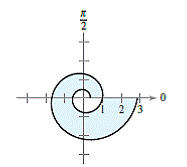Chapter 10.5, Problem 80E

Chapter
Section
Textbook Problem

# Logarithmic Spiral The curve represented by the equation r = a e b θ , where a and b are constants, is called a logarithmic spiral. The figure shows the graph of r = e θ / 6 , − 2 π ≤ θ ≤ 2 π . Find the area of the shaded region.To determine

To Calculate: The area of the shaded region.

Explanation

Given:

The curve represented by the equation r=aebθ; where a and b constants called logarithmic spiral the figures displayed in the graph of r=eθ6 and 2πθ2π.

Formula Used:

A=12αβ[f(θ)]2dθ

Calculation:

Area of spiral curve in the interval 2πθ2π will be

A=122π2πr2dθ

=122π2π(eθ6)2dθ

=122π2π

### Still sussing out bartleby?

Check out a sample textbook solution.

See a sample solution

#### The Solution to Your Study Problems

Bartleby provides explanations to thousands of textbook problems written by our experts, many with advanced degrees!

Get Started

#### In Exercises 1-6, simplify the expression. 1. x2+x2x24

Applied Calculus for the Managerial, Life, and Social Sciences: A Brief Approach

#### Rationalize each of the following denominators and simplify. (a) 5xy2x (b) yxxy23

Mathematical Applications for the Management, Life, and Social Sciences

#### Evaluate the integral. 30. x32x2+2x5x4+4x2+3dx

Single Variable Calculus: Early Transcendentals

#### The implied domain of is: (1, ∞) (−∞, 1) x ≠ 1 (−1, 1)

Study Guide for Stewart's Single Variable Calculus: Early Transcendentals, 8th